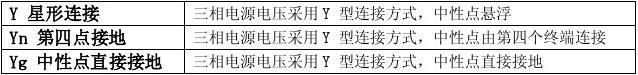# matlab的三相电源在哪,Matlab-Three-Phase Source(三相电源)

A 相初相角212base sc V L P f

π=? 基准电压

X/R 比值

2(/)/X fL R X R X R

π==